# Fourier transform, diffusion equation

Jack_O

## Homework Statement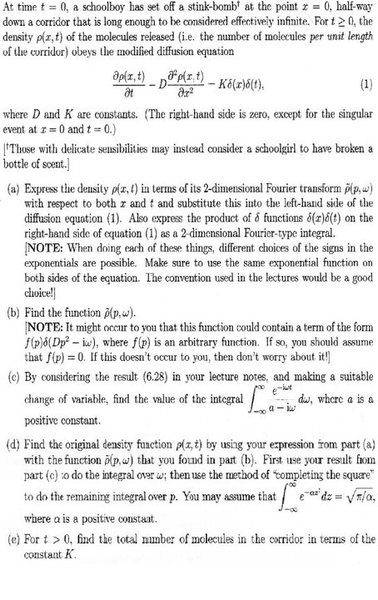## The Attempt at a Solution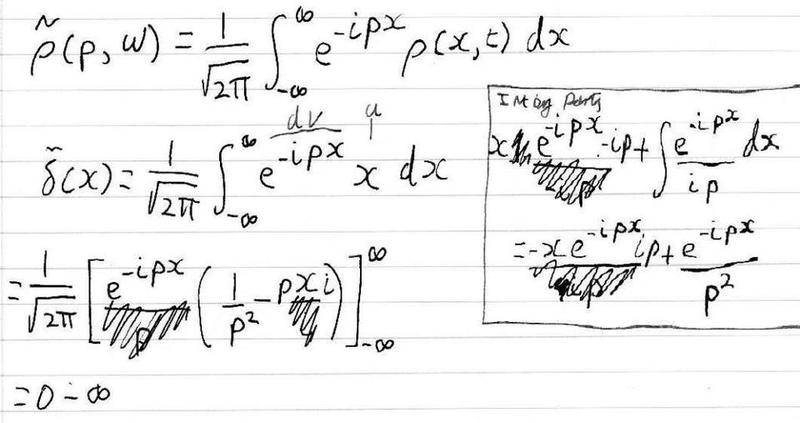I'm really at a loss on this question, which is why i have achieved so little on it so far. I think i more or less understand what a Fourier transform does (transpose amplitude vs time to amplitude vs frequency, ie the Fourier transform of two combined sine waves would be two spikes in frequency space).

In part a) I'm not sure how to express the product of d functions d(x)d(t) as a Fourier type integral.

Any help appreciated.

nickjer
Well that is a lot of questions, but let me help you on what you have done so far. First off, in your first line you forgot to take the Fourier transform of the time variable, yet you have $$\omega$$ in your function on the left hand side.

Also, for the delta functions since the variables are separated you can take the Fourier transform of each delta function individually. You tried to find the Fourier transform of $$\delta(x)$$, but forgot to include the delta function in the integral. For some reason you wrote it as x in the integral and not $$\delta(x)$$ which is very easy to integrate.

Jack_O
Ok I've had another go at this problem today, although i haven't really got any further than last time;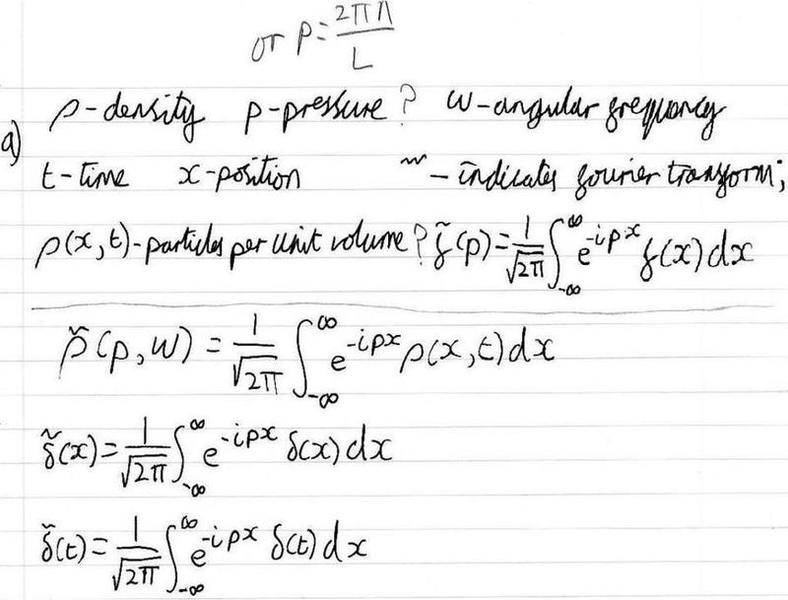I thought i had taken the Fourier transform of the time variable, as it is included in $$\rho$$(x,t)?

I am unsure what p (not rho) is supposed to represent in the question, at first i thought it was pressure due to the question being about diffusion in a gas, but after reading through my notes thoroughly it mentions p=(2$$\pi$$n)/L, and $$\delta$$p=(2$$\pi$$)/L when deriving the Fourier transform from the Fourier series. I don't see how that makes sense in this question though as L is infinite (as it has to be for the function to have a Fourier transform) which would mean p=0?

I'm not sure if i can take the integration any further than i have as their aren't given functions for $$\rho$$(x,t), $$\delta$$(x) or $$\delta$$(t).

Can i just sub the Fourier transforms as i have them now straight into the diffusion equation?

nickjer
Well I am used to seeing 'k' and not 'p'. 'k' is the wavenumber, k = 2*Pi/wavelength. So 'k' has units L-1.

Also you have set up the integrals for the dirac-delta functions, now you can solve them. They are very easy to solve. Although your last equation is incorrect... You should have 'omega' and 't' and not 'p' and 'x'.

Jack_O
Alright I've made a little more progress, I may have finished part a) but I'm not sure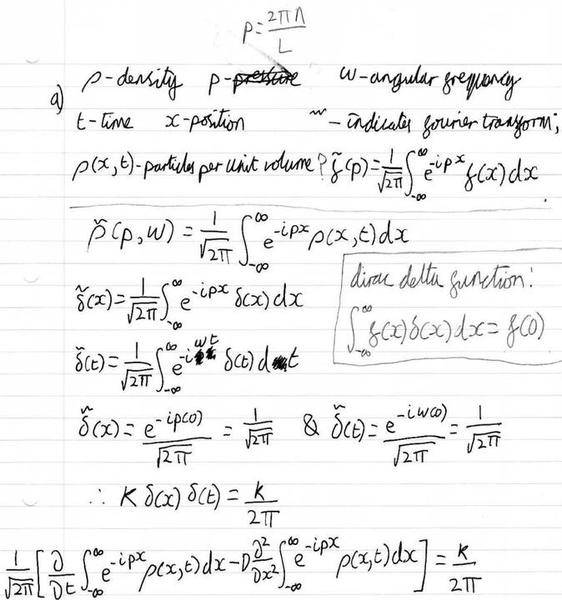I think replacing all the functions in the diffusion equation with their Fourier transforms means i effectively have the Fourier transform of the diffusion equation? I don't think i can cancel down the Fourier transform of $$\rho$$(x,t) at this point, which means i get a long equation when substituted into the diffusion equation.

Not got a clue about part b) be even if a) is correctThank you for your help so far nickjer.

nickjer
Well you got the transforms of the delta functions, but your final equation is incorrect. The first term should be:

$$\frac{\partial}{\partial t} \rho (x,t) = \frac{1}{2\pi} \frac{\partial}{\partial t} \int \int e^{+i(px+\omega t)} \tilde{\rho}(p,\omega) dp d\omega$$

So you can take the time derivative inside the integrals and get...

$$\frac{1}{2\pi} \int \int e^{+i(px+\omega t)} \cdot i\omega \tilde{\rho}(p,\omega) dp d\omega$$

Therefore the Fourier transform of the time derivative becomes:
$$\frac{\partial}{\partial t} \rho (x,t) \rightarrow i\omega \tilde{\rho}(p,\omega)$$

You can do the same for the 2nd term in your equation. Once you have done that, you can easily solve for $$\tilde{\rho}(p,\omega)$$ doing some algebra.

Last edited:
Jack_O
Ok i have taken the double differential for the position term now as well, in your last post you seem to suggest that once i have taken the differential i can forget about the integrals and exponential term, why is this?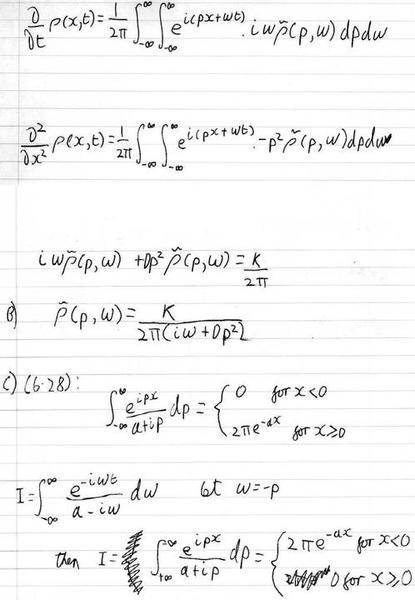Had a go at a bit more of the question, my answers still feel dodgy though.

nickjer
In (A), what is the object in front of the p^2? It should be a negative sign, but you seem to have a circle there. As for removing the integrals, well if you had done it to every term in your equation, then you'd have the exact same definite integrals everywhere. And they would cancel out, but too lazy to show it in detail.

Another way to think of it is you have...

$$\frac{\partial}{\partial t} \rho (x,t) = \frac{1}{2\pi} \int \int e^{+i(px+\omega t)} \cdot i\omega \tilde{\rho}(p,\omega) dp d\omega$$

Which is the exact same as...

$$f(x,t) = \frac{1}{2\pi} \int \int e^{+i(px+\omega t)} \tilde{f}(p,\omega) dp d\omega$$

Where under a Fourier transform f(x,t) goes to...

$$f(x,t) \rightarrow \tilde{f}(p,\omega)$$

comparing it to the first equation I listed you get the Fourier transform of d rho/dt as...

$$\frac{\partial}{\partial t} \rho (x,t) \rightarrow i\omega \tilde{\rho}(p,\omega)$$

Jack_O
In (A), what is the object in front of the p^2? It should be a negative sign, but you seem to have a circle there.

It's supposed to be a D (crappy handwriting), I've substituted each term back into the original equation given in the question, which is where the D comes from. The negative term i got for double position differential cancels with the negative term in the original equation i think.

nickjer
Alright then, I forgot about the -D in the original equation. So it looks good now.

As for (C), when you substituted w=-p, you forgot to substitute dw = -dp. Also, you are not using 'x' in that equation, you are using 't'.

Jack_O
Ok i get this for part c) now: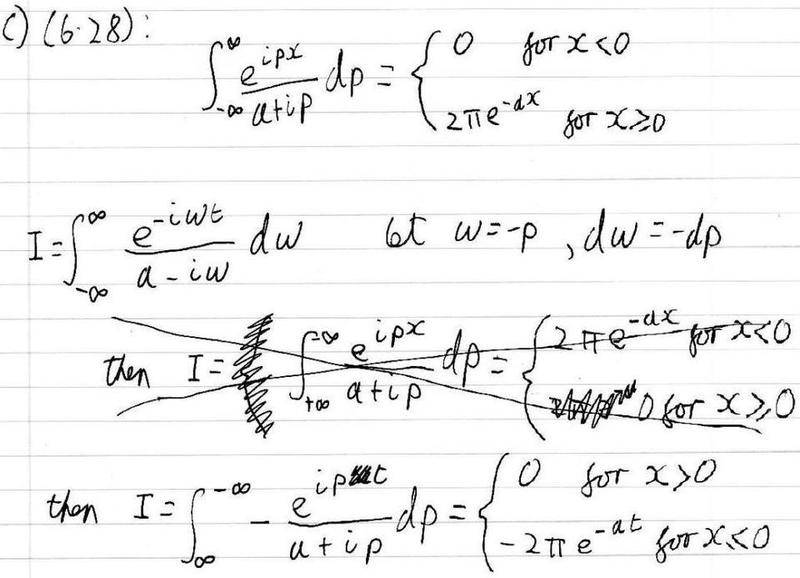Part d) doesn't seem to make sense now though, as i no longer have an integral over omega or p in my answer to a).

As for removing the integrals, well if you had done it to every term in your equation, then you'd have the exact same definite integrals everywhere. And they would cancel out

Unless i can't remove all the integrals because when i used the dirac delta funtion to evaluate the RHS of the equation it removed the integrals from RHS but not the LHS, so perhaps the integrals on the LHS should have remained?

nickjer
(D) is just asking for rho(x,t). So do the inverse Fourier transform of rho(p,omega) that you solved for in (B).